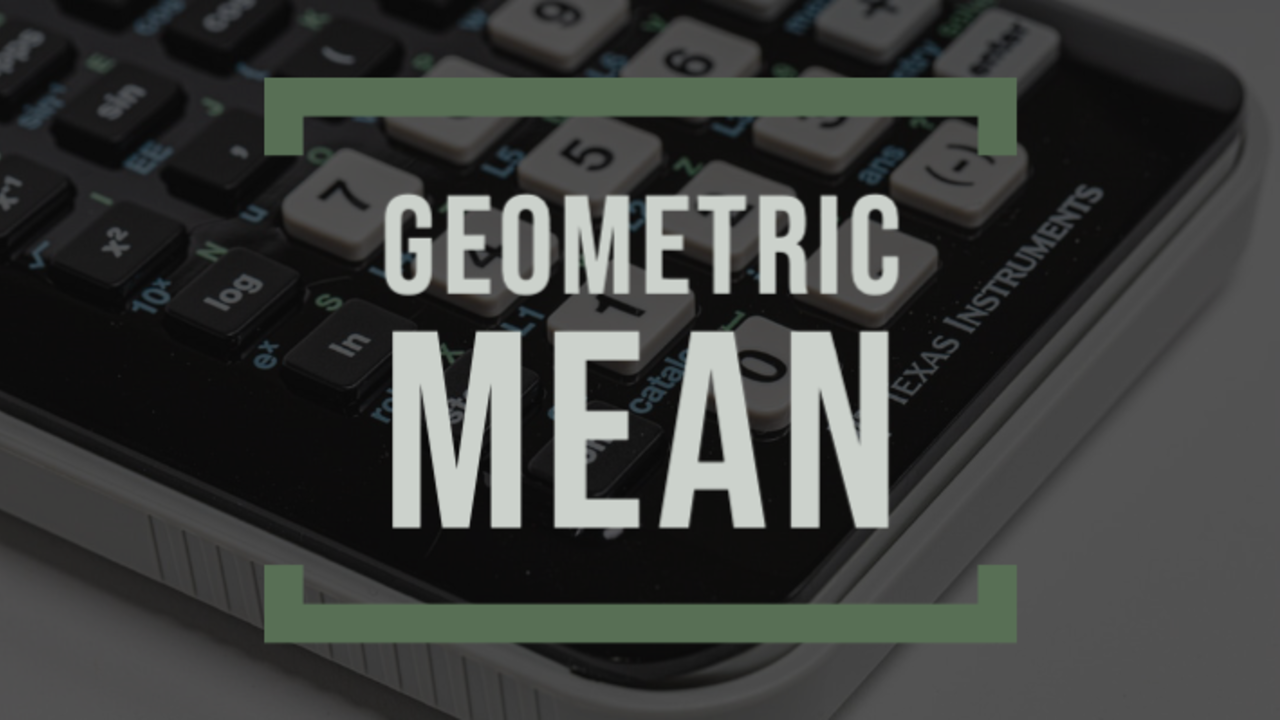# GEOMETRIC MEAN

Mar 11, 2020Definition
The geometric mean is the average of a set of numbers.

Why It's Important
This is not to be confused with arithmetic mean.  The arithmetic mean is what you learned in school when your teacher taught you about "mean".  As a refresher, let's look at the following set of numbers: 3%,7%,10%,6%,4%.  You find the mean by adding all the numbers together (3+7+10+6+4=30%) then dividing by the total number of records (5).  The arithmetic mean in this scenario would be 6%.  In contrast, a geometric mean would be calculated as (1.03x1.07x1.10x1.06x1.04)^(1/5)=~5.5015%.

Now that you have the concept, let's apply it to personal finance and investing.  The geometric mean is typically used to calculate the average return on investments.  You would look at the past annual rates and calculate the mean to find out what your overall return was over the time period.

For example, let's say one year you enjoyed 10%, the next 15%, and the next 8%.  Your average return over the three years would be (1.10x1.15x1.08)^(1/3)=~10.627%!

Here is a Geometric Mean Calculator

For any of You Math Nerds , Its Formula iswhere n is the total number of values and xi (x2, x1, ... ,xn) are the individual numbers in the data set. The formula is equivalent to:In other words: It's the nth root of the product of n values.

## Stay connected with news and updates!

Close